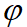Hill Publishing Group | contact@hillpublisher.com

#Location：Home / Journals / Journal of Applied Mathematics and Computation /

DOI：http://dx.doi.org/10.26855/jamc.2023.03.021

# Error Bounds for Krylov Approximation and Exact Solution of Identifying an Unknown Source in the Poisson Equation

Date: May 6,2023 |Hits: 1229 Download PDF How to cite this paper

Ousmane Samba Coulibaly1,*, Boureima Sangaré2

1Department of Mathematics, University of Science, Techniques and Technologies of Bamako (USTTB), Bamako, Mali.

2Department of Mathematics, University Nazi BONI, Bobo-Dioulasso, Houet, Burkina Faso.

*Corresponding author: Ousmane Samba Coulibaly

### Abstract

In this paper, we treat an ill-posed problem for the determination of an unknown source in the Poisson equation. We make a theoretical analysis of the approximation of the function(A)g = (I −e −A) −1A2g by using the Krylov subspace me-thod, and we derive some error estimates. The idea is to project the approximate operator (I − e −A) −1A 2 on a small subspace, and perform matrix calculations that result. Having good estimates or even bounds for the error in computing approximations to expression of the f(A)v is very important in pratical application. This opportunity is for us to propose a basic approach to solving the inverse problem, then estimate the error to see its effectiveness and the convergence of the solution. This general approach, which has been used with success in several applications, provides a systematic way of defining high order explicit-type schemes for solving of Poisson equation.

### References

 A. Alqahtani, R. Ramlau, L. Reichel. Error Estimates for GolubKahan Bidiagonalization with Tikhonov Regularization for Illposed Operator Equations, Abdulaziz Alqahtani et al 2022 Inverse Problems in press https://doi.org/10.1088/1361-6420/aca754, pp. 1- 23.

 Abdulaziz Alqahtani, Silvia Gazzola, Lothar Reichel, Giuseppe Rodriguez. On the block Lanczos and block GolubKahan reduction methods applied to discrete ill-posed problems, Wiley, Numer Linear Algebra Appl. 2021;e2376. wileyonlineli-brary.com/journal/nla, pp. 1- 22.

 Ou Xie, Zhenyu Zhao. Identifying an unknown source in the Poisson equation by a modified Tikhonov regularization method, International Journal of Mathematical and Computational Sciences 6 (2012), pp. 86- 90.

 Nicholas J. Higham, Matrix Functions, Theory and Application, SIAM - Philadelphia, USA, 2008.

 V. Druskin and L. Knizhnerman, Extended Krylov subspaces: approximation of the matrix square root and related functions, SIAM J. Matrix Anal. Appl. 19 (1998), 755-771.

 V. Druskin and L. Knizhnerman, Krylov subspace approximation of eigenpairs and matrix functions in exact and computer arithmetic, Numer Linear Algebra Appl. 2 (1995), 205- 217.

 Y.Saad , Iterative Methods for Sparse Linear Systems, second ed., SIAM - Philadelphia, USA, 2003 .

 X.X.Li, F.Yang,The truncation method for identifying the heat source dependent on a spatial variable, Computers and Mathematics with Applications 62 (2011), pp. 2497- 2505.

 Monaghan, A.J., Chebyshev series approximation on complex domains, Durham theses, Durham University. Available at Durham E-Theses Online: http://etheses.dur.ac.uk/7168/, 1984.

 V. L. Druskin and L. A. Knizhnerman, Two Polynomial methods of calculating functions of symmetric matrices, U.S.S.R comput.maths.math.phys., vol.29, No.6 (1989), pp. 112-121.

 Stefan Paszkowski, Computation applications of Chebyshev polynomials and series, Moscow, Nauka, 1983.

 Abdallah Bouzitouna, Nadjib Boussetila, Faouzia Rebbani, Two regularization methods for a class of inverse boundary value problems of elliptic type, Bouzitouna et al. Boundary Value Problems 2013, 2013:178, pp. 1. 23. a SpringOpen Journal http://www.boundaryvalueproblems.com/content/2013/1/178.

 I. Moret and P. Novati, Krylov subspace methods for functions of fractional differential operators. Mathematics of Computation, 88, 11 2017. doi: 10.1090/mcom/3332.

 M. Benzi and I. Simunec, Rational Krylov methods for fractional diffusion problems on graphs. arXiv e-prints, art. ar-Xiv:2012.08389, dec 2020.

 Stefan G¨uttel, Convergence Estimates of Krylov Subspace Methods for the Approximation of Matrix Functions Using Tools from Potential Theory, Masters thesis, Technische Universit¨at Bergakademie Freiberg 2006.

### How to cite this paper

Error Bounds for Krylov Approximation and Exact Solution of Identifying an Unknown Source in the Poisson Equation

How to cite this paper: Ousmane Samba Coulibaly, Boureima Sangaré. (2023) Error Bounds for Krylov Approximation and Exact Solution of Identifying an Unknown Source in the Poisson EquationJournal of Applied Mathematics and Computation7(1), 188-201.

DOI: http://dx.doi.org/10.26855/jamc.2023.03.021

## More Journals